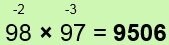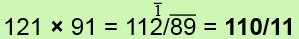# Vedic Math-3 Multiplications

## Vedic Math-How to Multiply 2 Digit Numbers ?

### Vedic Math Rules : Multiplication of NumbersThe deficiencies (12 and 2) have been written above the numbers (on the flag), the minus signs indicating that the numbers are below 100.

The answer 8624 is in two parts: 86 and 24.
The 86 is found by taking one of the deficiencies from the other number.
That is:  88 – 2 = 86 or 98 – 12 = 86 (whichever you like), and the 24 is simply the product of the deficiencies: 12 × 2 = 24.The differences from 100 are 7 and 4,
93 – 4 = 89 or 96 – 7 = 89,
and 7 × 4 = 28.Here the numbers are each 11 below 100, and 11 × 11 = 121, a 3-figure number. The hundreds digit of this is therefore carried over to the left.

### 568 × 998 = 566864

We see here that the numbers 568 and 998 are conveniently close to 1000, so we allow 3 figures on the right. The differences of the numbers from 1000 are 432 and 2.

The method is just the same:
568 – 2 = 566,
432 × 2 = 864.

However in the case of 568 here the deficiency is not so obvious as in the previous examples, and this is exactly where the Sutra of the present chapter comes in.

### Vedic Math : If All From 9 and the Last From 10 is applied to the digits of 568 we get 432:

take the 5 from 9 and get 4,
take the 6 from 9 and get 3,
we take the 8 from 10 and get 2.

This formula gives the deficiency of any number from the next highest base. It could have been applied in the previous examples too: for 88, in the first example, we take the first 8 from 9 to get 1, and the last from 10 to get 2. This gives the deficiency of 12 below the base of 100. For 98 we get 02, or just 2.

### 58776 × 99998 = 58774/82448

58776 – 2 = 58774, and the formula gives the deficiencies 41224, 2 which are multiplied together to get 82448.

Similarly 857 × 994 = 851858

where 851 = 857 – 6, and 858 = 143 × 6.

103 × 104 = 10712

This is even easier than the previous examples, but the method is just the same. The deficiencies are +3 and +4: positive now because the numbers are above the base.

103 + 4 = 107 or 104 + 3 = 107, and 4 × 3 = 12.

12 × 13 = 156 (12+3=15, 2×3=6)

1234 × 1003 = 1237702 (1234+3=1237, 234×3=702)

10021 × 10002 = 100230042 (10021+2=10023, 0021×2=0042)

124 × 98 = 12248 = 12152

Here the base is 100 and the deficiencies from 100 are +24 and -2.

Applying the usual procedure we find 124 – 2 = 122 or 98 + 24 = 122.
So 122 is the left-hand part of the answer.
Then multiplying the deficiencies we get -48, written 48 (since a plus times a minus gives a minus). This gives the answer as 12248.
To remove the negative portion of the answer we just take 48 from one of the hundreds in the hundreds column. This simply means reducing the hundreds column by 1 and applying All From 9 and the Last From 10 to 48. Thus 122 becomes 121 and 48 becomes 52.
So 12248 = 12152.

### 1003 × 987 = 990/039 = 989/961

Similarly, we first get 1003-13 = 990 or 987+3 = 990,
and +3 × -13 = 039 (3 figures required here as the base is 1000).
Then 990 is reduced by 1 to 989, and applying the formula to 039 gives 961.Here we have a minus one to carry over to the left so that the 112 is reduced by 2 altogether.

213 × 203 = 2 × 216/39 = 43239

We observe here that the numbers are not near any of bases used before: 10, 100, 1000 etc. But they are close to 200, with deficiencies of 13 and 3.
The usual procedure gives us 216/39 (213+3=216, 13×3=39).
Now since our base is 200 which is 100×2 we multiply only the left-hand part of the answer by 2 to get 43239.

### 29 × 28 = 3×27/2 = 812

The base is 30 (3×10), and the deficiencies are -1 and -2.
29-2 = 27, 1×2 = 2 and 3×27 = 81.

311 × 298 = 3×309/22 = 926/78

Here the numbers are above and below 300: we multiply the left-hand side by 3 before deducting 1 to deal with the negative right-hand portion.

### 88 × 49 = half of (88×98) = half of (8624) = 4312

9998 × 94 = 9398/12

The bases here are 10,000 and 100 and the deficiencies are -2 and -6.
The answer is in two parts: 9398 and 12.
In subtracting the deficiency 6 from the first number, imagine the numbers are under each other:

9998
94

and subtract the 6 in the column indicated by the last figure of the smaller number, that is, the second column from the left here.
So 9998 becomes 9398.
Then multiply the deficiencies together: 2×6 = 12.

1032 × 98 = 1012/64 = 101136

### Vedic Math :  Note here that because 98 = 102 the deficiency 2 is deducted from the 3 to give 1012 on the left.

98 × 97 × 96 = 91/26/24 = 912576

These numbers are all close to 100, their deficiencies being 2, 3, 4.
The answer is in 3 parts as indicated by the oblique lines.
First take one number and take both of the other deficiencies from it.
For example 98-3-4 = 91. This is the first part of the answer.

Then multiply the deficiencies together in pairs and add the results up:
2×3 + 2×4 + 3×4 = 6+8+12 = 26.
This is the middle part of the answer.

Finally just multiply all 3 deficiencies together: 2 × 3 × 4 = 24.
We write 24 because the deficiencies are actually negative.
The 24 is then removed as in Examples 13 and 14 earlier.

1022 × 1002 × 1003 = 1027/116/132

1022+2+3 = 1027, 22×2 + 22×3 + 2×3 = 116, 22×2×3 = 132

### 962 = 92/16

96 is 4 below 100, so we reduce 96 by 4, which gives us the first part of the answer. The last part is just 4= 16 as the formula says.

### 10062 = 1012/036

Here 1006 is increased by 6 to 1012, and 62 = 36: but with a base of 1000 we need 3 figures on the right, so we put 036.

### 3042 = 3×308/16 = 92416

This is the same but because our base is 300 the left-hand part of the answer is multiplied by 3.

Note the following alternative method: if we look at the number split so that
3042 = 9/24/16, then we may see that 9 = 32,
24 = twice 3 × 4, and 16 = 42.

### 542 = 29/16

Since 502 = 2500 and we have 542, which is 4 more,
we have 25 + 4 = 29,
and 42 = 16.

482 = 2304

25 – 2 = 23 (25, as before, minus the deficiency, 2)
22 = 4 (the deficiency squared)

## Multiplication By Nines

The Vedic formula By One Less Than the One Before, which is the converse of the formula of the previous chapter, comes in here in combination with All From 9 and the Last From 10.

763 × 999 = 762/237

The number being multiplied by 9’s is first reduced by 1:763-1 = 762, then All From 9 and the Last From 10 is applied to 763 to get 237.

1867 × 99999 = 1866/98133

Here, as 1867 has 4 figures, and 99999 has 5 figures, we suppose 1867 to be 01867. This is reduced by 1 to give 1866,
and applying All From 9…. to 01867 gives 98133.

Vedic math Tips and techniques are useful for all students of Age 8-15 years.